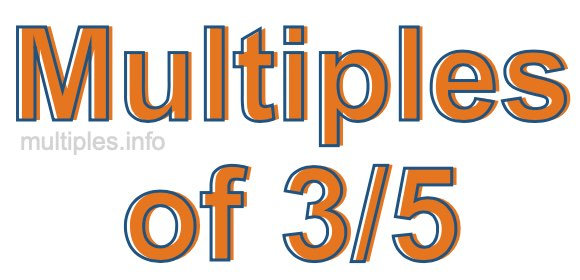Multiples of 3/5We define the multiples of 3/5 as the product of any integer multiplied by 3/5. Therefore, to create a list of multiples of 3/5 we start by multiplying 3/5 by one, then we multiply 3/5 by two, then we multiply 3/5 by three, and so on.

To multiply a fraction by an integer, we leave the denominator alone and multiply the numerator by the integer. It is impossible to give you a list of all multiples of 3/5 because the list goes on forever. However, here is the math to calculate the beginning list of multiples of 3/5.

3/5 × 1 = 3/5

3/5 × 2 = 6/5

3/5 × 4 = 9/5

3/5 × 4 = 12/5

3/5 × 5 = 15/5

3/5 × 6 = 18/5

3/5 × 7 = 21/5

3/5 × 8 = 24/5

3/5 × 9 = 27/5

3/5 × 10 = 30/5

3/5 × 11 = 33/5

3/5 × 12 = 36/5

We have simplified the fractions above for you. Below are the multiples of 3/5 in their lowest possible terms:

3/5 = 3/5

6/5 = 1 1/5

9/5 = 1 4/5

12/5 = 2 2/5

15/5 = 3

18/5 = 3 3/5

21/5 = 4 1/5

24/5 = 4 4/5

27/5 = 5 2/5

30/5 = 6

33/5 = 6 3/5

36/5 = 7 1/5

As we stated above, the list of multiples of 3/5 goes on forever because the product of 3/5 multiplied by any whole number (integer) is a multiple of 3/5. You can also create a list of multiples of 3/5 if you keep adding 3/5 like this:

3/5
3/5 + 3/5 = 6/5
3/5 + 3/5 + 3/5 = 9/5
3/5 + 3/5 + 3/5 + 3/5 = 12/5

Again, the list can go on forever, because you can continue adding 3/5 to the list. Therefore, the list of multiples of 3/5 is ∞ (infinite).

Multiples of Fractions
Do you need the multiples of another fraction? Enter a another fraction below to get the multiples of that fraction.

/

Multiples of 3/6
Go here for the next fraction on our list that we have multiples for.